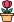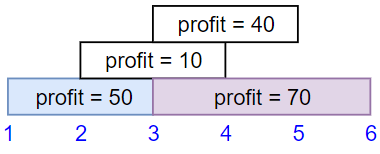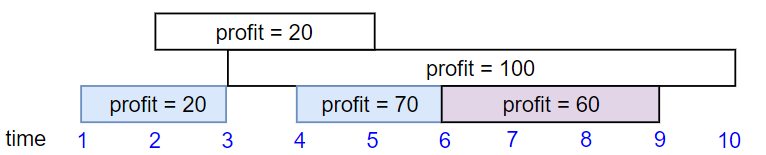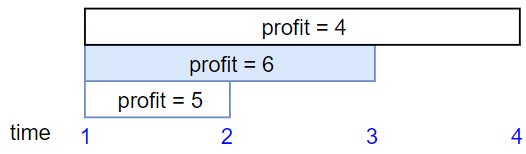엔지니어 게시판
LeetCode 솔루션 분류

# [11/26] 1235. Maximum Profit in Job Scheduling

### 작성자 정보

•작성
• 작성일

• 222 조회
• 1 댓글
• 0 추천
• 0 비추천

### 본문

Hard

We have `n` jobs, where every job is scheduled to be done from `startTime[i]` to `endTime[i]`, obtaining a profit of `profit[i]`.

You're given the `startTime``endTime` and `profit` arrays, return the maximum profit you can take such that there are no two jobs in the subset with overlapping time range.

If you choose a job that ends at time `X` you will be able to start another job that starts at time `X`.

Example 1:```Input: startTime = [1,2,3,3], endTime = [3,4,5,6], profit = [50,10,40,70]
Output: 120
Explanation: The subset chosen is the first and fourth job.
Time range [1-3]+[3-6] , we get profit of 120 = 50 + 70.
```

Example 2:```Input: startTime = [1,2,3,4,6], endTime = [3,5,10,6,9], profit = [20,20,100,70,60]
Output: 150
Explanation: The subset chosen is the first, fourth and fifth job.
Profit obtained 150 = 20 + 70 + 60.
```

Example 3:```Input: startTime = [1,1,1], endTime = [2,3,4], profit = [5,6,4]
Output: 6
```

Constraints:

• `1 <= startTime.length == endTime.length == profit.length <= 5 * 104`
• `1 <= startTime[i] < endTime[i] <= 109`
• `1 <= profit[i] <= 104`
Accepted
168,543
Submissions
315,081

댓글 1

## 학부유학생님의 댓글

• 익명
• 작성일
Runtime: 1631 ms, faster than 30.10% of Python3 online submissions for Maximum Profit in Job Scheduling.
Memory Usage: 27.4 MB, less than 65.43% of Python3 online submissions for Maximum Profit in Job Scheduling.
``````import bisect
class Solution:
def jobScheduling(self, startTime: List[int], endTime: List[int], profit: List[int]) -> int:
jobs = sorted(zip(startTime,endTime, profit), key=lambda x:x)

dp = [[0,0]]

for s,e,p in jobs:
i = bisect.bisect_left(dp, [s+1])-1
if dp[i] + p > dp[-1]:
dp.append([e, dp[i]+p])

return dp[-1]``````
전체 349 / 1 페이지

• 등록일 02.01
• 등록일 02.01
• 등록일 02.01
• 등록일 02.01
• 등록일 02.01
• 등록일 02.01
• 등록일 02.01
• 등록일 02.01
• 등록일 02.01
• 등록일 02.01
• 등록일 02.01
• 등록일 02.01

• 등록일 01.31
• 등록일 01.31
• 등록일 01.31
• 등록일 01.27
• 등록일 01.27
• 등록일 01.23
• 등록일 01.23

### Stats

• 현재 접속자 90 명
• 오늘 방문자 99 명
• 어제 방문자 1,716 명
• 최대 방문자 2,853 명
• 전체 회원수 535 명